Interactive Lecture Demonstration

Prediction Sheet—Rotational Motion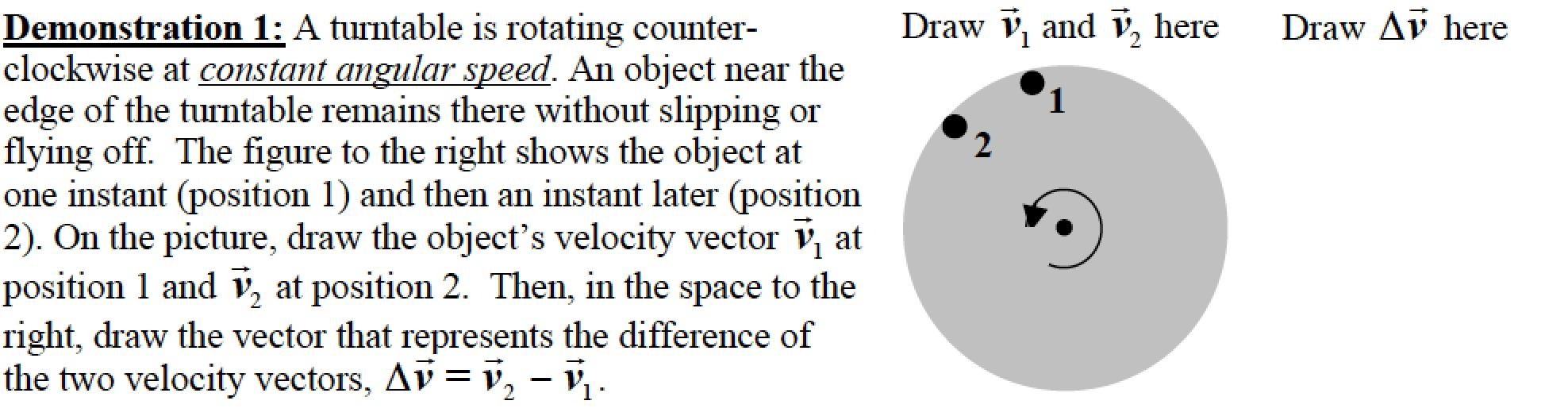Only after you have made your predictions, open the simulation Ladybug Revolution: https://phet.colorado.edu/sims/cheerpj/rotation/latest/rotation.html?simulation=rotation Drag the ladybug to the outside edge. Use the slider to set the Angular Velocity to about 60 rad/s. Observe the velocity and acceleration as the bug passes through position 1 and position 2. Compare the two velocities to your predictions and explain any differences.   What is the direction of the acceleration relative to the center of the disk?   Click here to see the vector subtraction. Compare the result to your prediction. Describe the direction of the vector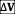relative to the center of the disk. How does this compare to the direction of the acceleration? Explain why. Demonstration 2: For the motion of the turntable described in Demonstration 1, sketch on the diagram on the right the net force acting on the object at the edge of the turntable when it is at position 1. What is the cause of this force?   Only after you have made your prediction, click here to view the force. Compare the force to your prediction, and explain why the force must be in this direction to cause the described motion.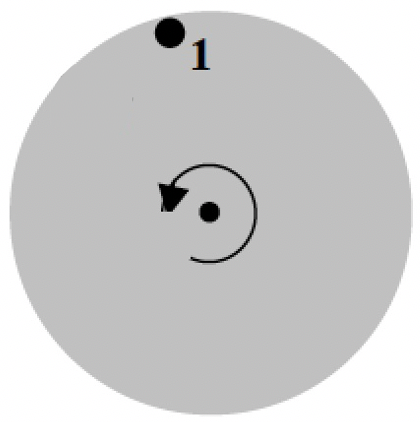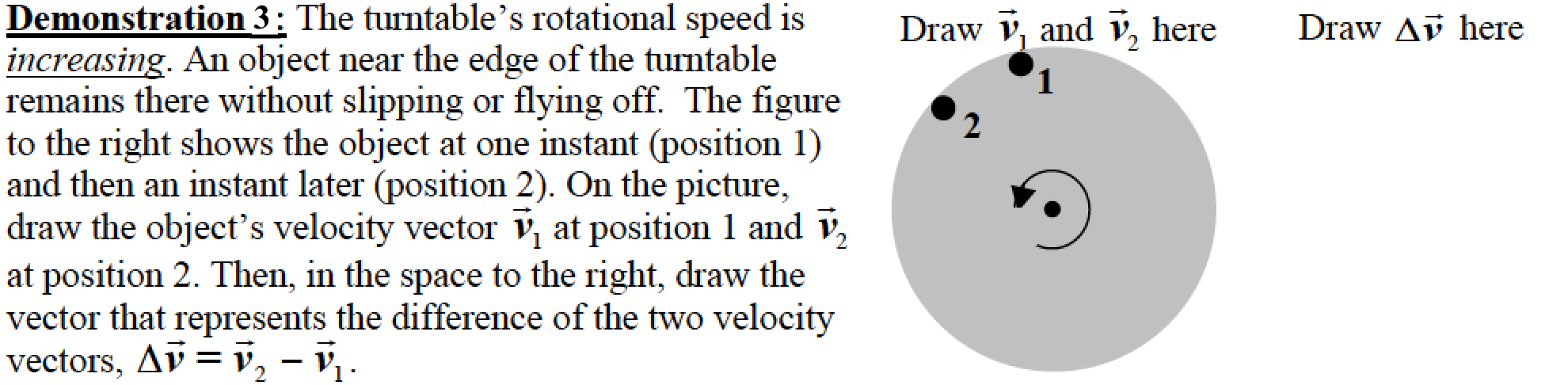Only after you have made your predictions click here to see the two velocity vectors and the vector subtraction. Compare the two velocities to your predictions and explain any differences.   Describe the direction of the vectorrelative to the center of the turntable.   Now use the same Ladybug Revolution simulation.  The ladybug should be on the edge of the turntable in position 1. Place the cursor on the left edge of the turntable, quickly rotate the turntable counterclockwise, and observe the direction of the acceleration as the ladybug is accelerating towards position 2. Describe the direction of a and compare it to the direction of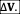Explain what you observe. Demonstration 4: For the motion of the disk described in Demonstration 3, sketch on the diagram on the right the net force acting on the object at the edge of the turntable when it is at position 1. What is the cause of this force?   Only after you have made your prediction, click here to view the force. Compare the force to your prediction, and explain why the force must be in this direction to cause the described motion.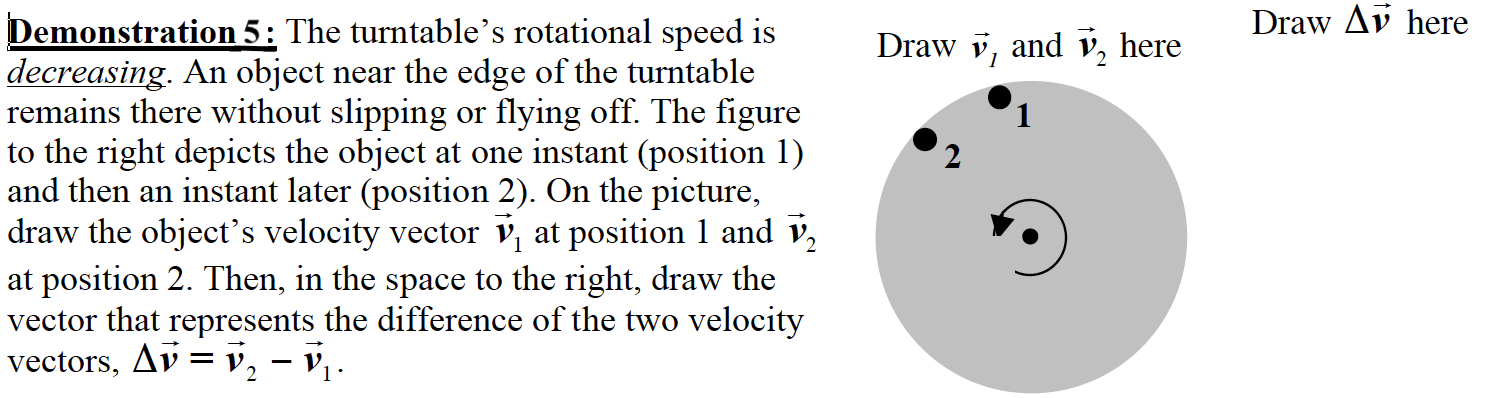Only after you have made your predictions click here to see the two velocity vectors and the vector subtraction. Compare the two velocities to your predictions and explain any differences.   Describe the direction of the vectorrelative to the center of the turntable.   Now use the same Ladybug Revolution simulation.  The ladybug should be on the edge of the turntable in position 1. Place the cursor on the left edge of the turntable, start rotating the turntable counterclockwise, and observe the direction of the acceleration as you slow the turntable. Be sure that you are observing a at a moment when it is slowing down. Describe the direction of a and compare it to the direction ofExplain what you observe. Demonstration 6: For the motion of the disk described in Demonstration 5, sketch on the diagram on the right the net force acting on the object at the edge of the turntable when it is at position 1.   Only after you have made your prediction, click here to view the force. Compare the force to your prediction, and explain why the force must be in this direction to cause the described motion.